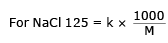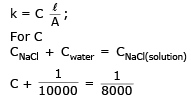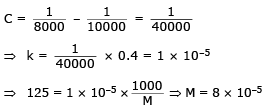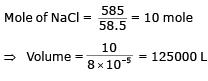JEE  >  Electrochemistry MCQ - 1 (Advanced)

# Electrochemistry MCQ - 1 (Advanced)

Test Description

## 19 Questions MCQ Test Chemistry for JEE Advanced | Electrochemistry MCQ - 1 (Advanced)

Electrochemistry MCQ - 1 (Advanced) for JEE 2023 is part of Chemistry for JEE Advanced preparation. The Electrochemistry MCQ - 1 (Advanced) questions and answers have been prepared according to the JEE exam syllabus.The Electrochemistry MCQ - 1 (Advanced) MCQs are made for JEE 2023 Exam. Find important definitions, questions, notes, meanings, examples, exercises, MCQs and online tests for Electrochemistry MCQ - 1 (Advanced) below.
 1 Crore+ students have signed up on EduRev. Have you?
*Multiple options can be correct
Electrochemistry MCQ - 1 (Advanced) - Question 1

### During discharging of lead storage battery, which of the following is/are true?

Detailed Solution for Electrochemistry MCQ - 1 (Advanced) - Question 1

Pb(s) + PbO2 + 2H2SO4 → 2PbSO1 (s) + 2H2O
H2SO4 : consumed
H2O : produced (volume increases and density decreases)
Anode
Pb (S) + SO42+ → PbSO4 + 2e
Cathode
2e + 4H+ + PbO2 + So42– → PbSO4 (s) + 2H2O

*Multiple options can be correct
Electrochemistry MCQ - 1 (Advanced) - Question 2

### Which of the following arrangement will produce oxygen at anode during electrolysis?

Detailed Solution for Electrochemistry MCQ - 1 (Advanced) - Question 2

(A) At anode oxidation of Cu produce Cu2+
(B) At both electrode oxidation or reduction of hydrogen or H+
(C) At anode
4OH → O2 + 2H2O + 4e
(D) Anode
2H2O → 4H+ + O2 + 4e

*Multiple options can be correct
Electrochemistry MCQ - 1 (Advanced) - Question 3

### If 270.0 g of water is electrolysed during an experiment performed by miss abhilasha with 75% current efficiency then

Detailed Solution for Electrochemistry MCQ - 1 (Advanced) - Question 3

Cell reaction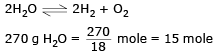equivalent = 15 × 2 = 30 equivalent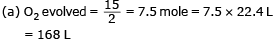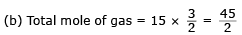Total volume of gas produced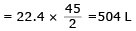(c) H2 produced = 30 × 22.4 = 336 L
at cathode current efficilency = 75%
(d) for 30 F electricity consumed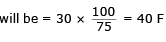*Multiple options can be correct
Electrochemistry MCQ - 1 (Advanced) - Question 4

Pick out the correct statements among the following from inspection of standard reduction potentials (Assume standard state conditions).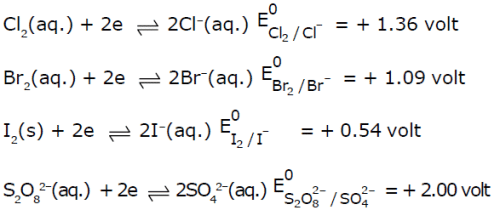Detailed Solution for Electrochemistry MCQ - 1 (Advanced) - Question 4

(a) SOP (SO42–) = –2.00
SOP (Cl) = – 1.36
oxidation of SO42– will not take place
(b) SOP (Cl) = – 13.6
SOP (I) = –0.54
SOP (Br) = –1.09
(c) Similarly as (b) option
(d) Br can’t be reduced further.

*Multiple options can be correct
Electrochemistry MCQ - 1 (Advanced) - Question 5

The EMF of the following cell is 0.22 volt.

Ag(s) |AgCl(s)|KCl(1M)|H+(1M)|H2(g) (1atm); Pt(s)

Which of the following will decrease the EMF of cell.

Detailed Solution for Electrochemistry MCQ - 1 (Advanced) - Question 5

Cell reaction
Anode
Ag(s) + Cl–1 → AgCl (s) + e
Cathode 2H+ + 2e → H2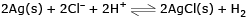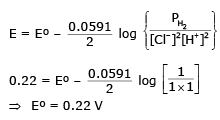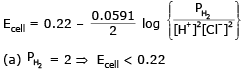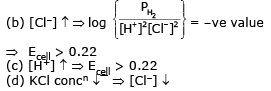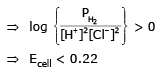Electrochemistry MCQ - 1 (Advanced) - Question 6

Equal volumes of 0.015 M CH3COOH & 0.015 M NaOH are mixed together. What would be molar conductivity of mixture if conductivity of CH3COONa is 6.3 × 10-4 S cm-1

Detailed Solution for Electrochemistry MCQ - 1 (Advanced) - Question 6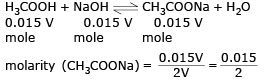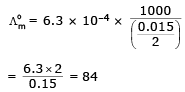Electrochemistry MCQ - 1 (Advanced) - Question 7

The questions given below consist of Statement – I and Statement – II. Use the following Key to choose the appropriate answer.

Statement-1 : The voltage of mercury cell remains constant for long period of time.

Statement-2 : It is because net cell reaction des not involve active species.

Detailed Solution for Electrochemistry MCQ - 1 (Advanced) - Question 7

HgO Cathode   ;  Zn (anode)
Zn → Zn2+ + 2e
Hg2+ + 2e → Hg
Cell reaction
Zn + HgO → ZnO + Hg
No active species in cell reactions
⇒ no change in cell voltage.

Electrochemistry MCQ - 1 (Advanced) - Question 8

The questions given below consist of Statement – I and Statement – II. Use the following Key to choose the appropriate answer.

Statement-1 : The SRP of three metallic ions A+, B2+, C3+ are -0.3, -0.5, 0.8 volt respectively, so oxidising power of ions is C3+ > A+ > B2+

Statement-2 : Higher the SRP, higher the oxidising power.

Detailed Solution for Electrochemistry MCQ - 1 (Advanced) - Question 8

SRP increases  ⇒  The tendency to getting reduced increases and the oxidizing power increases.

Electrochemistry MCQ - 1 (Advanced) - Question 9

The questions given below consist of Statement – I and Statement – II. Use the following Key to choose the appropriate answer.

Statement-1 : We can add the electrode potential in order to get electrode potential of net reaction.

Statement-2 : Electrode potential is an intensive property.

Detailed Solution for Electrochemistry MCQ - 1 (Advanced) - Question 9

We can add only when the net reaction does not contain any electron [E.P. is an intensive property]

Electrochemistry MCQ - 1 (Advanced) - Question 10

The questions given below consist of Statement– I and Statement – II. Use the following Key to choose the appropriate answer.

Statement - I. In electrolysis, the quantity of electricity needed for depositing 1 mole of silver is different form that required for 1 mole of copper.

Statement - II. The atomic weights of silver and copper are different.

Detailed Solution for Electrochemistry MCQ - 1 (Advanced) - Question 10

Statement I : is correct as the n factor of both silver and copper are different so the gm equivalent of electricity required will be different .
Statement II : It is also correct that atomic weight of siliver and copper are different but statement II is not the reight explanation of I. So answer (B).

Electrochemistry MCQ - 1 (Advanced) - Question 11

The questions given below consist of Statement – I and Statement – II. Use the following Key to choose the appropriate answer.

Statement - I. CuSO4 solution cannot safely be kept in a Zn vessel usual.

Statement - II. The position of Zn is lower than Cu in Electro-chemical-series.

Detailed Solution for Electrochemistry MCQ - 1 (Advanced) - Question 11

Statement I : is correct beause copper lies at higher position in electrichenical sesier so upper will start deposition in vessel.
Statement II : It is false because copper is at higher position so Ans (C)

Electrochemistry MCQ - 1 (Advanced) - Question 12

The questions given below consist of Statement – I and Statement– II. Use the following Key to choose the appropriate answer.

Statement - I. Zinc displaces copper from copper sulphate solution..

Statement - II. The Eº is Zn of –0.76 volt and that of copper is +0.34 volt.

Detailed Solution for Electrochemistry MCQ - 1 (Advanced) - Question 12

Statement I  is correct because
Zn + CuSO4 → Cu + ZnSO4 Zinc oxidation takes place and reduction of copper take blake.
Statement II : Is also correct and given values are correct So Ans. (A)

Electrochemistry MCQ - 1 (Advanced) - Question 13

The questions given below consist of Statement – I and Statement – II. Use the following Key to choose the appropriate answer.

Statement - I. Gold chloride (AuCl3) solution cannot be stored in a vessel made of copper, iron, nickel, chromium, zinc or tin.

Statement - II. Gold is very precious metal.

Detailed Solution for Electrochemistry MCQ - 1 (Advanced) - Question 13

Statement I : It is correct as gold has higher reduction potential.
Statement II : It is also correct but not the correct explanation of I.

Electrochemistry MCQ - 1 (Advanced) - Question 14

The questions given below consist of Statement – I and Statement – II. Use the following Key to choose the appropriate answer.

Statement - I . Specific conductance decreases with dilution whereas equivalent conductance increases.

Statement - II. On dilution, number of ions per cc decreases but total number of ions increases considerably.

Detailed Solution for Electrochemistry MCQ - 1 (Advanced) - Question 14

Statement I : It is correct
λ = KV
K = λ/V
thus inversely proportional.
Statement II :  It is also correct because number of ions per CC decreases and also correct explation of I.

Electrochemistry MCQ - 1 (Advanced) - Question 15

The questions given below consist of Statement – I and Statement – II. Use the following Key to choose the appropriate answer.

Statement - I. Salt bridge is used in Electro chemical cell.

Statement - II. The ions of the electrolyte used in salt bridge should have almost same ionic mobility.

Detailed Solution for Electrochemistry MCQ - 1 (Advanced) - Question 15

Statement I : It is correct
Statement II : It is correct but not the correct explanation of above

Electrochemistry MCQ - 1 (Advanced) - Question 16

The questions given below consist of Statement – I and Statement – II. Use the following Key to choose the appropriate answer.

Statement - I. Absolute value of an electrode potential can not be calculated.

Statement - II. Neither oxidation nor reduction can take place alone.

Detailed Solution for Electrochemistry MCQ - 1 (Advanced) - Question 16

Statement I : Assolute value can’t be calculated it is only calculated W.r.t. hydrogen.
Statement II : It is wrong

Electrochemistry MCQ - 1 (Advanced) - Question 17

Comprehension

Paragraph -1

A sample of water from a large swimming pool has a resistance of 10000 ohm at 25ºC when placed in a certain conductance cell. When filled with 0.02 M KCl solution, the cell has a resistance of 100 ohm at 25ºC. 585 gm of NaCl were dissolved in the pool, which was throughly stirred. A sample of this solution gave a resistance of 8000 Ω.

[Given : Molar conductance of NaCl at that concentration is 125 ohm-1cm2mol-1 and molar conductivity of KCl at 0.02 M is 200 Ω-1cm2 mol-1.]

Q.

Cell constant (in cm-1) of conductance cell is :

Detailed Solution for Electrochemistry MCQ - 1 (Advanced) - Question 17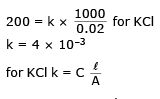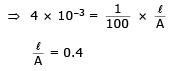Electrochemistry MCQ - 1 (Advanced) - Question 18

A sample of water from a large swimming pool has a resistance of 10000 ohm at 25ºC when placed in a certain conductance cell. When filled with 0.02 M KCl solution, the cell has a resistance of 100 ohm at 25ºC. 585 gm of NaCl were dissolved in the pool, which was throughly stirred. A sample of this solution gave a resistance of 8000 Ω.

[Given : Molar conductance of NaCl at that concentration is 125 ohm-1cm2mol-1 and molar conductivity of KCl at 0.02 M is 200 Ω-1cm2 mol-1.]

Q.

Conductivity (Scm-1) of H2O is :

Detailed Solution for Electrochemistry MCQ - 1 (Advanced) - Question 18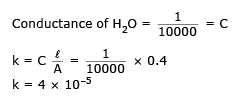Electrochemistry MCQ - 1 (Advanced) - Question 19

A sample of water from a large swimming pool has a resistance of 10000 ohm at 25ºC when placed in a certain conductance cell. When filled with 0.02 M KCl solution, the cell has a resistance of 100 ohm at 25ºC. 585 gm of NaCl were dissolved in the pool, which was throughly stirred. A sample of this solution gave a resistance of 8000 Ω.

[Given : Molar conductance of NaCl at that concentration is 125 ohm-1cm2mol-1 and molar conductivity of KCl at 0.02 M is 200 Ω-1cm2 mol-1.]

Q.

Volume (in Litres) of water in the pool is:

Detailed Solution for Electrochemistry MCQ - 1 (Advanced) - Question 19